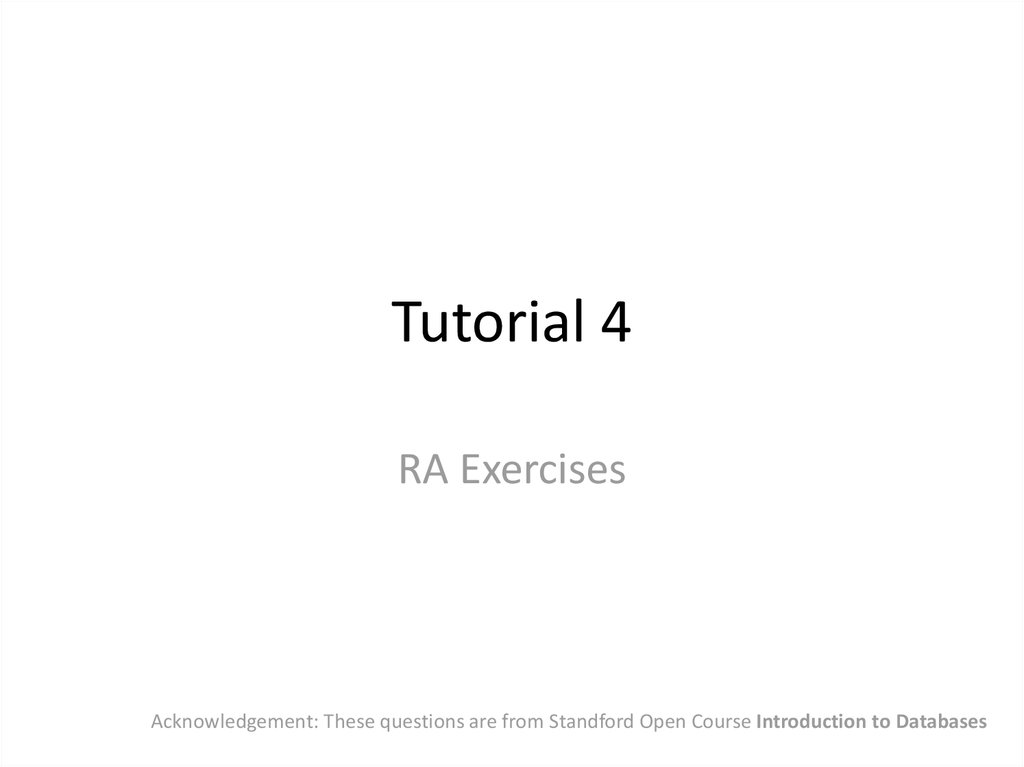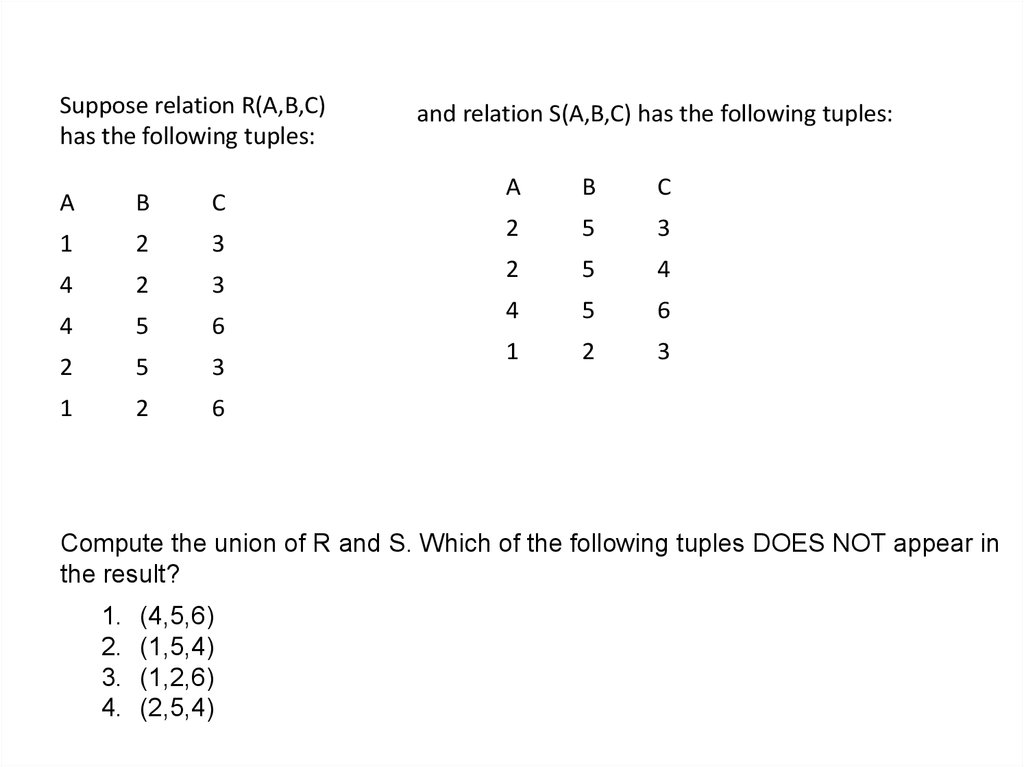# Acknowledgement: These questions are from Standford Open Course Introduction to Databases

## 1. Tutorial 4

RA Exercises
Acknowledgement: These questions are from Standford Open Course Introduction to Databases

## 2. Compute the union of R and S. Which of the following tuples DOES NOT appear in the result?

Suppose relation R(A,B,C)
has the following tuples:
A
B
C
1
2
3
4
2
3
4
5
6
2
5
3
1
2
6
and relation S(A,B,C) has the following tuples:
A
B
C
2
5
3
2
5
4
4
5
6
1
2
3
Compute the union of R and S. Which of the following tuples DOES NOT appear in
the result?
1.
2.
3.
4.
(4,5,6)
(1,5,4)
(1,2,6)
(2,5,4)

## 3.

Suppose relation R(A,C) has the
following tuples:
A
C
3
3
6
4
2
3
3
5
7
1
and relation S(B,C,D) has the following
tuples:
B
C
D
5
1
6
1
5
8
4
3
9
Compute the natural join of R and S. Which of the following tuples is
in the result? Assume each tuple has schema (A,B,C,D).
1.
2.
3.
4.
(6, 4, 3, 9)
(3, 3, 5, 8)
(7, 1, 5, 8)
(3, 1, 5, 8)
To compute the natural join, we must find
tuples from R and S that agree on all common
attributes. In this case, C is the only attribute
appearing in both schemas, and the tuples in
the join result have attributes A, B, C, and D -the union of the attributes from R and S.

## 4.

Suppose relation R(A,B) has the following tuples:
A
B
1
2
3
4
5
6
and relation S(B,C,D) has the following tuples:
B
C
D
2
4
6
4
6
8
4
7
9
Compute the theta-join of R and S with the condition R.A < S.C AND R.B < S.D.
Which of the following tuples is in the result? Assume each tuple has schema (A,
R.B, S.B, C, D).
1. (3,4,4,6,8)
2. (3,4,4,7,8)
3. (3,4,5,7,9)
4. (1,2,2,6,8)

## 5.

Suppose relation R(A,B,C) has the following tuples:
A
B
C
1
2
3
4
2
3
4
5
6
2
5
3
1
2
6
and relation S(A,B,C) has the following tuples:
A
B
C
2
5
3
2
5
4
4
5
6
1 2 3
Compute (R - S) union (S - R), often called the "symmetric difference" of R
and S. Which of the following tuples is in the result?
1. (4,5,3)
2. (2,5,3)
3. (4,5,6)
4. (2,5,4)

## 6.

• Consider a relation R(A) with r tuples, all
unique within R, and a relation S(A) with s
tuples, all unique within S. Let t represent the
number of tuples in R minus S. Which of the
following triples of values (r,s,t) is possible?
1.
2.
3.
4.
(10,5,2)
(5,3,1)
(5,0,3)
(5,3,4
R minus S has at most r tuples (if no values of R
are also in S) and as few as max(r-s,0) tuples (if
all values of R are also in S).